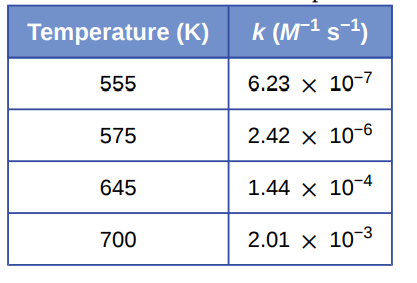# Problem: Hydrogen iodide, HI, decomposes in the gas phase to produce hydrogen, H2, and iodine, I2. The value of the rate constant, k, for the reaction was measured at several different temperatures and the data are shown here:What is the value of the activation energy (in kJ/mol) for this reaction?

###### FREE Expert Solution

We need to use the two-point form of the Arrhenius Equation:

where:

k = rate constant

Ea = activation energy (in J/mol)

R = gas constant (8.314 J/mol • K)

T = temperature (in K)

A = Arrhenius constant or frequency factor

81% (48 ratings)###### Problem Details

Hydrogen iodide, HI, decomposes in the gas phase to produce hydrogen, H2, and iodine, I2. The value of the rate constant, k, for the reaction was measured at several different temperatures and the data are shown here:What is the value of the activation energy (in kJ/mol) for this reaction?

Frequently Asked Questions

What scientific concept do you need to know in order to solve this problem?

Our tutors have indicated that to solve this problem you will need to apply the Arrhenius Equation concept. You can view video lessons to learn Arrhenius Equation. Or if you need more Arrhenius Equation practice, you can also practice Arrhenius Equation practice problems.

What professor is this problem relevant for?

Based on our data, we think this problem is relevant for Professor Lewis' class at UGA.

What textbook is this problem found in?

Our data indicates that this problem or a close variation was asked in Chemistry - OpenStax 2015th Edition. You can also practice Chemistry - OpenStax 2015th Edition practice problems.